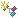﻿ Multiple Axes.netCHARTING v9.2 Documentation Send comments on this topic. Multiple Axes
 Getting Started > General Tutorials > Axis Tutorials > Multiple Axes

Glossary Item Box

Multiple Axes

.netCHARTING employs a straightforward yet effective system to utilize multiple axes. Each series can have it's own x and y axis. By default each series on a chart inherits the main x and y axis

Main Axes

• Chart.YAxis
• Chart.XAxis

Axes are defined for series using the following properties:

• Series.XAxis
• Series.YAxis

The relationships and identification of axes is maintained by axis object instances. This means instead of specifying an axis name you would specify it by using the axis object itself.

For example, here both series will use the same x axis:

```[C#]
Series1.XAxis = new Axis();Series2.XAxis = Series1.XAxis;```
```[Visual Basic]
Series1.XAxis = New Axis()Series2.XAxis = Series1.XAxis```

This example will show how two different axes are specified for two series.

```[C#]
DataEngine de = new DataEngine();//(... get data into the engine ...)SeriesCollection sc = de.GetSeries();// Let's assume the data engine generated 2 series.// Specify different axes for each series.sc.XAxis = new Axis();sc.YAxis = new Axis();sc.XAxis = new Axis();sc.YAxis = new Axis();// Add the series collection to the chart.Chart.SeriesCollection.Add(sc);
```
```[Visual Basic]
Dim de As New DataEngine()'(... get data into the engine ...)Dim sc As SeriesCollection = de.GetSeries()' Let's assume the data engine generated 2 series.' Specify different axes for each series.sc(0).XAxis = New Axis()sc(0).YAxis = New Axis()sc(1).XAxis = New Axis()sc(1).YAxis = New Axis()' Add the series collection to the chart.Chart.SeriesCollection.Add(sc)```Samples: RateChart.aspx SeriesAxisBinding.aspx SeriesAxisBindingDB.aspx InvisibleAxis.aspx

See also: Z Axis effect using multiple axes